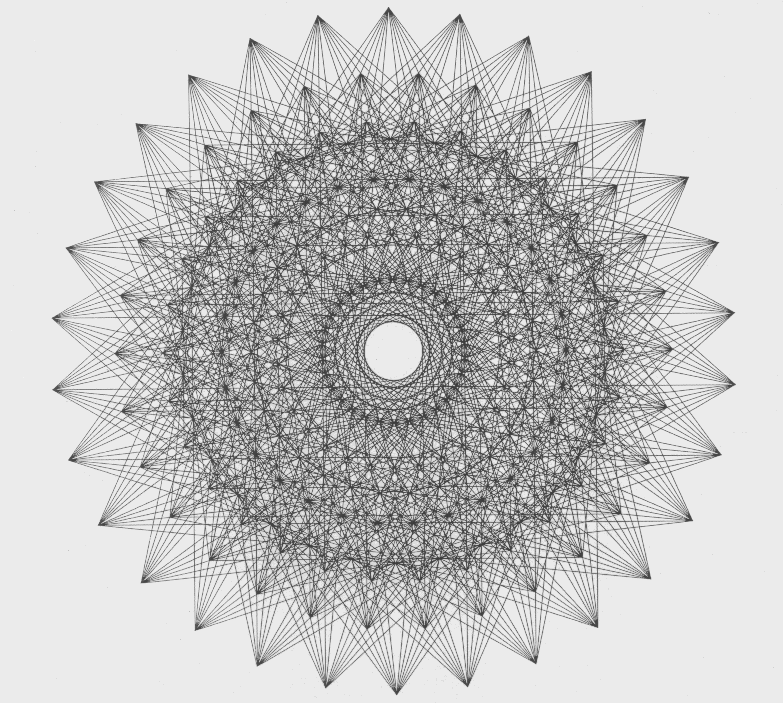## Euclidean geometry

I find that I come across this subject quite a lot in my research and didn’t really have a clear understanding of it. So it’s time we did a bit of digging.

Now I usually go to Wikipedia first because even though it might not be 100% accurate – and sometimes any random person might have added the information – on well known topics, there is less room for mistakes, as others will be checking these too and making corrections if necessary. However, I find that it’s harder to grasp – maybe my brain isn’t on full form tonight.

I like this explanation from the University of Toronto’s Mathematics Network – http://www.math.toronto.edu/mathnet/questionCorner/euclidgeom.html:

Euclidean geometry is just another name for the familiar geometry which is typically taught in grade school: the theory of points, lines, angles, etc. on a flat plane. It is given the name “Euclidean” because it was Euclid who first axiomatized it (rigorously described it).

Another reason it is given the special name “Euclidean geometry” is to distinguish it from non-Euclidean geometries (described in the answer to another question).

The difference is that Euclidean geometry satisfies the Parallel Postulate (sometimes known as the Fifth Postulate). This postulate states that for every line l and every point p which does not lie on l, there is a unique line l‘ which passes through p and does not intersect l (i.e., which is parallel to l).

Geometry on a curved surface, for example, may not satisfy this postulate, and hence is non-Euclidean geometry.

But what are the other postulates then?

So I search again and find this brief explanation instead on QR Glossary:

Euclidean Geometry is one of many different types of geometries. It is the most familiar one, typically studied in high school (and never again). All of the theorems and conclusions of Euclidean Geometry can be derived from these five basic postulates:

1. A straight line segment can be drawn joining any two points.

2. Any straight line segment can be extended indefinitely in a straight line.

3. Given any straight line segment, a circle can be drawn having the segment as radius and one endpoint as center.

4. All right angles are congruent.

5. If two lines are drawn which intersect a third in such a way that the sum of the inner angles on one side is less than two right angles, then the two lines inevitably must intersect each other on that side if extended far enough.

The fifth postulate is equivalent to what is known as “The Parallel Postulate.” Another way to state The Parallel Postulate is to say: Given a line(l) and a point(P) not on that line, there is exactly one line which can be drawn through P that is parallel to l. Non-Euclidean geometries are created by failing to accept The Parallel Postulate. These include Hyperbolic Geometry and Spherical Geometry, among others.

I don’t remember this from school! It might have made more sense otherwise. Ok I think diagrams are needed. And also a comparison to show the different types of geometry.

This image is pretty easy to understand and makes the difference between the two clearer:http://www.mathreference.com/geo,intro.html

This seems like an easy site to pick up a bit of knowledge from about this subject. I don’t want to just copy and paste it – so read up if you want all this to make more sense.

I’m planning to add to this topic soon. Especially once I’ve read up on the other types of geometry and can then hopefully speak about each without having to use someone else’s words 🙂

In the brief search I just conducted online I also came across this image:http://www.math.toronto.edu/gif/polytope.gif

I find it absolutely beautiful. I feel like dismissing everything else and just jump straight to finding out more about Polytopes!! But I won’t – not just yet. I need to grasp a strong understanding of the basics and the levels leading to the higher more complicated levels before I do so.

Explore posts in the same categories: Uncategorized